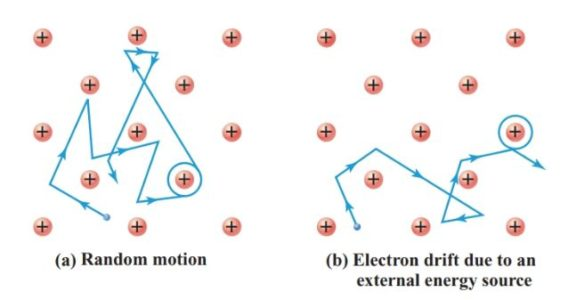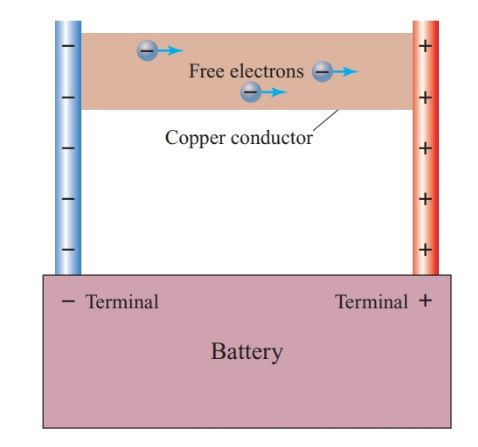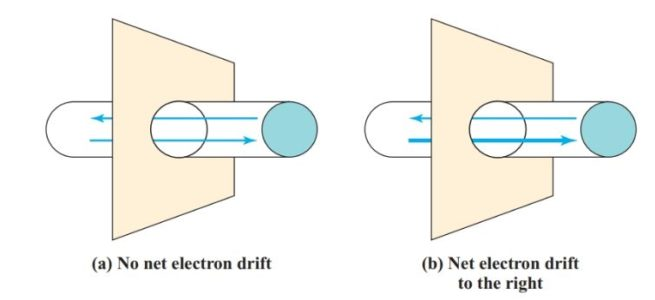# What is Electric Current? Definition, Unit, Formula & Examples

Want create site? Find Free WordPress Themes and plugins.

An electric current is a flow of charged particles. In most circuits, these charged particles are free electrons.

Although there is a free electron for each copper ion, Figure 1 shows only one of these free electrons so that we may trace its motion through the lattice.

In Figure 1(a), this free electron moves randomly from atom to atom. The random motion of all of the free electrons in the conductor averages out, so there is no net flow of electrons in any direction.

Figure 1(b) shows the same conductor when connected in an electric circuit that conveys energy to the free electrons. This external source of energy superimposes a net electron drift to the right on the random motion of the free electrons.Figure 1          Motion of a free electron in a copper conductor

The battery in Figure 2 adds electrons to the left end of the conductor and, at the same time, removes the same number of electrons from the right end of the conductor.

The battery uses chemical energy to maintain a surplus of electrons (a net negative charge) at its negative terminal, and a deficiency of electrons (a net positive charge) at its positive terminal.

The free electrons in the conductor are repelled by the negative charge at the negative terminal of the battery and are attracted by the positive charge at the positive terminal of the battery. Since these electrons are free to move from atom to atom in the lattice of copper ions, the result is a net electron drift from left to right in the conductor.Figure 2          Motion of free electrons due to energy from a battery

To determine the net movement of free electrons in an electric conductor, we can consider an imaginary plane cutting across the conductor at right angles to its length.

Figure 3(a) shows the conductor with no external source of energy applied to it. In any given time, the number of electrons crossing the plane in one direction equals the number crossing in the opposite direction. There is no net electron drift, and, hence, no electric current.

In Figure 3(b), an external energy source causes more electrons to cross the imaginary plane from left to right than from right to left. Consequently, there is now a net electron drift to the right. This net drift of charge carriers is an electric current.Figure 3          Net movement of electrons in a conductor

## Electric Current Definition

Electric current is the net flow of charge carriers past a given point in an electric circuit in a given period of time.

In an electric circuit, the current from the energy source to the load is equal to the current from the load back to the energy source.

The Coulomb

Since each electron possesses the same elemental quantity of charge, we may express the net electron drift across the imaginary plane in Figure 3 in terms either of the number of electrons or of the total charge possessed by this number of electrons.

A practical unit for expressing the quantity of electric charge must represent the charge carried by many billions of electrons.

The coulomb (symbol C) is the SI unit of quantity of electric charge. A coulomb is the quantity of electric charge carried by 6.24 × 1018 electrons.

The letter symbol for the quantity of electric charge is Q, while the letter e represents the charge on one electron.

## Electric Current Example 1

How much charge is carried by 2.40 × 1019 free electrons?

Solution

$Q=\frac{2.40\times {{10}^{19}}e}{6.24\times {{10}^{18}}{}^{e}/{}_{C}}=3.85C$

## Electric Current Formula & Unit

Electric current is measured in terms of the rate of charge flow. The SI unit of electric current, the ampere, is named after a French pioneer of electrical physics, André Marie Ampère (1775–1836).

Unit: The ampere (symbol A) is the SI unit of electric current.

One ampere is equal to a flow of one coulomb of charge per second:

1 A = 1 C/s.

The letter symbol for electric current is I.

Formula: The relationship between current and charge is

$I=\frac{Q}{t}$

Where I is current in amperes, Q is charge in coulombs, and t is a time in seconds.

## Electric Current Example 2

Find the current in an electric heater when 75 C of charge pass through the heater in half a minute.

Solution

\begin{align} & \begin{matrix} I=\frac{Q}{t}= & \frac{75C}{30s}= & 2.5{}^{C}/{}_{s} & 2.5A \\\end{matrix} \\ & \begin{matrix} \uparrow & {} & \begin{matrix} {} & \uparrow & \begin{matrix} {} & {} & \uparrow & \begin{matrix} {} & {} & \uparrow \\\end{matrix} \\\end{matrix} \\\end{matrix} \\\end{matrix} \\ & \begin{matrix} step1 & step2 & {} & \begin{matrix} step3 & {} & step4 & {} \\\end{matrix} \\\end{matrix} \\ \end{align}

When using a calculator, we tend to simply enter the data to get a numerical answer in a single step. However, a systematic approach is often helpful, especially for more complex calculations. Here is the sequence of steps for solving Example 2.

Step 1

Note that the problem states two pieces of information and asks for one. To express this information in equation form, write the symbol for the unknown quantity on the left of the equals sign and the symbols for the given data in their proper relationship on the right of the equals sign.

Step 2

Substitute the given data into the equation, making sure that powers of 10 or unit prefixes are included to preserve the proper magnitude.

Step 3

Perform the numerical computation.

Step 4

The calculator displays the magnitude of the answer in root units. Express the answer in appropriate units, using unit prefixes if necessary.

## Electric Current Example 3

How long will it take 4.0 mC of electric charge to pass through a fuse if the current is 50 A?

Solution

Since,

$I=\frac{Q}{t}$

$t=\frac{Q}{I}=\frac{4mC}{50{}^{C}/{}_{s}}=8\times {{10}^{-5}}s=80ms$

## Conventional Current and Electron Flow

The charge carriers in metallic conductors are free electrons which flow from the negative terminal of the voltage source toward the positive terminal. However, the electric current can also result from the flow of positive and negative ions.

For calculating the algebraic sum of the currents at a junction in a circuit, we need to define the direction of current flow in a way that we can apply to all types of charge carriers.

Since most circuit conductors are metal, it would be logical to define the current direction in terms of electron flow. However, experimenters established the convention for current direction long before the electron was discovered.

Since Michael Faraday (1791–1867) showed that metals transfer from the positive terminal to the negative terminal in electrolytic cells, the direction of conventional current was taken to be from positive to negative. Positive charge carriers flow in the opposite direction to negative charge carriers such as free electrons and negative ions.

Therefore, the laws and calculations for electric circuits were based on the current flowing from the positive terminal of the source, through the circuit, and back to the negative terminal.

So, we need to keep in mind that conventional current is simply a mathematical convention for describing current and that electrons flow in the opposite direction.

The circuit diagrams in this text show the direction of conventional current.

Did you find apk for android? You can find new Free Android Games and apps.